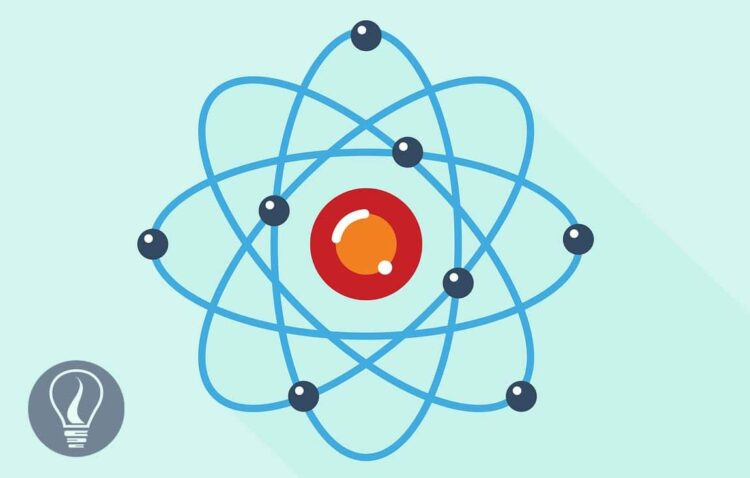More results...

Generic selectors
Exact matches only
Search in title
Search in content
Post Type Selectors

# Introduction to Atomic TheoryB.Sc (Hons, USJ) (Polymer Science and Technology, Chemistry, Physics)
Categories
Last Updated On : January 19, 2023
Published Date : August 8, 2021

Atom is the smallest building block of a substance that shows the identity of that substance. This was first proposed by Democritus.

## Atomic Theory

Atomic Theory describes that matter is composed of atoms. Electrons, protons, and neutrons are the building units of the atom. The studies and works of Galileo, Newton, Maxwell, and other physicists were very useful in the discovery of the atom and the building of atomic theory.

Around 1890, most physicists and chemists thought that matter was built from atoms. It was widely believed that atoms cannot divide further into smaller units. The “gas laws” and the “law of definite proportions (Proust's law)” were the evidence for this model of the atom. The “law of definite proportions” states that, when a compound is completely divided into its basic units, the masses of the constituent always have the same ratio.

It is now known that all the elements were composed of three different types of particles (subatomic particles). Those subatomic particles are Electrons, Protons, and Neutrons.

Electrons carry a negative charge (–e), Protons carry a positive charge (+e) and Neutrons do not carry a net electrical charge. The mass of the neutron is approximately the same as the proton. To understand the structure of the atom, ideas of quantum theory needed to be applied.

## Atomic models

An atomic model theoretical representation that describes the structure of an atom. A number of atomic models have been introduced based on experimental evidence, but the exact structure of the atom is still quite unknown.

### 01. Plum Pudding Model

Sir Joseph John Thomson (1856-1940) also known as J.J. Thomson was a British physicist who discovered the electron which was the first subatomic particle to be discovered. He introduced the first atomic model that was the Plum Pudding Model. The basic idea of the Plum pudding model is that the positive charge of the atom is distributed as a “pudding” with electrons suspended throughout the “pudding” like plums in the pudding.

### 02. Rutherford’s atomic model

A neutral atom has zero total electric charges. Therefore, an atom must contain a precise amount of positive “pudding” in order to neutralize the electrons, according to the Plum Pudding Model. How was that accomplished? So, physicists studied how atoms collide with other atomic-scale particles. These experiments were carried out by New Zeeland physicist, Ernest Rutherford (1871-1937), German physicist Hans Geiger (1882-1945), and New Zeeland physicist Ernest Marsden (1889-1970). Rutherford’s atomic model or Planetary model proposed the evidence-based gold foil experiment.

A beam of α particles that was emitted by a radioactive source was focused on a thin section of gold foil. Most of the α particles travel through the foil undeflected. That means the atom has the most empty spaces. Some of the α particles (positively charged particles) were deflected by small angles. A small number of alpha particles were deflected through very large angles. Some were bounced backward.

The plum pudding model couldn’t explain the reaction of the alpha particle. Then Rutherford realized that all the positive charges in an atom must be concentrated in a very small volume. Both mass and the density of the positive charge on the atom were equal to the alpha particle. Most of the alpha particles missed this dense area of the atom and passed through the atom. Sometimes an alpha particle collided with a dense area, and then it showed a large deflection. Then Rutherford came to the conclusion that atoms have a “nucleus” that is positively charged and the nucleus has a much higher mass than the electrons.

Rutherford then suggested that the atom is kind of like a smaller version solar system. The electrons of the atom orbit around the nucleus just like the planets orbit the sun in the solar system. The electrons must move in orbits to avoid falling into the nucleus because of the electric force. The atomic nucleus contains protons, and the proton has a positive charge (+e). Because the total charge on an atom is equal to zero, the number of protons in the nucleus of an atom must equal the number of electrons present in that atom.

The major problem with the Planetary Model is the stability of the electron orbit. Atoms are stable. But, according to the planetary model, atoms should not be stable. It was impossible to make the atom stable in the planetary model. Electrons should emit electromagnetic radiation because of their undergoing accelerated motion. Because an electron loses energy as electromagnetic radiation, it should travel as a spiral toward the nucleus as the below figure. So, according to the planetary model, the atom would be unstable, and atoms should only last a fraction of a second.

## Quantum Theory Solution for atomic model

Quantum theory avoids the problem of unstable electrons. It replaces orbits with discrete energy levels. Quantum theory states that electrons are not simple particles that obey Newton’s laws and spiral into the nucleus. The electron is defined as a wave-particle described by a “wave function” with separate, individual energy levels. The only time Electrons gain or lose energy is when electrons go through a transition between energy levels.